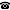# 2009 seminar talk: Constructive Reverse Mathematics

Talk held by Douglas S. Bridges (University of Canterbury, New Zealand) at the KGRC seminar on 2009-01-22.

### Abstract

Reverse mathematics is a program in mathematical logic that seeks to determine which axioms are required to prove theorems of mathematics. Constructive reverse mathematics is reverse mathematics carried out in Bishop-style constructive math (BISH)---that is, using intuitionistic logic and, where necessary, constructive ZF set theory. There are two primary foci of constructive reverse mathematics:

• first, investigating which constructive principles are necessary to prove a given constructive theorem;
• secondly, investigating what non-constructive principles are necessary additions to BISH in order to prove a given non-constructive theorem.

I will introduce basic ideas of constructive mathematics and constructive reverse mathematics, paying particular attention to connections between various continuity properties, versions of the fan theorem, and a simple principle due to Ishihara.

Kurt Gödel Research Center for Mathematical Logic. Währinger Straße 25, 1090 Wien, Austria.+43-1-4277-50501. Last updated: 2010-12-16, 04:37.